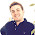Wednesday, August 22, 2012

Find the minimum distance

Given an unsorted array arr[] and two numbers x and y, find the minimum distance between x and y in arr[]. The array might also contain duplicates. You may assume that both x and y are different and present in arr[]

1.function minimumDistance (arr[],X,Y)
{
Lung=length(arr[]); //Lunghezza array
PX=Lung; //posizione di X
PY=Lung; //posizione di Y
DXY=Lung; //distanza XY
for(i=0, i abs(PX-PY)) DXY=abs(PX-PY); //Aggiorna la distanza minima
}
}
return DXY;
}

*meglio che calcolare tutte le distanze, O(Lungh)

2.Just an idea:

Build a map of positions of each unique element in arr[]:

map[a] = list of positions in arr[] where "a" appears.

This require O(n).

Then, generate all the pairs of elements from the lists map[x] and map[y]. Return the pair (a, b) where abs(a - b) - 1 is minimum.

Maybe the pair generation is not the optimum but keep the algo simple to implement :)